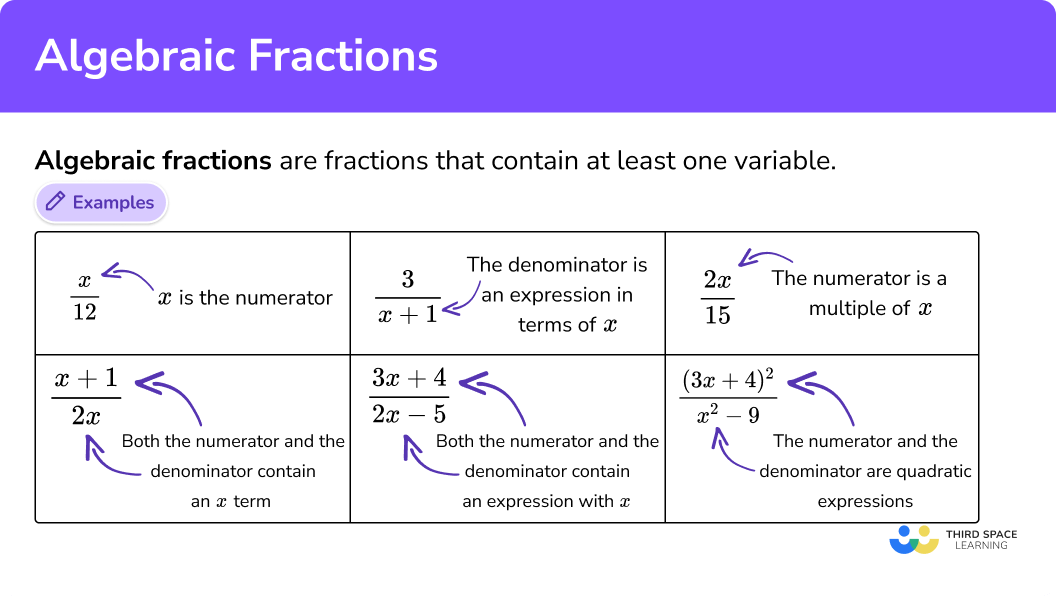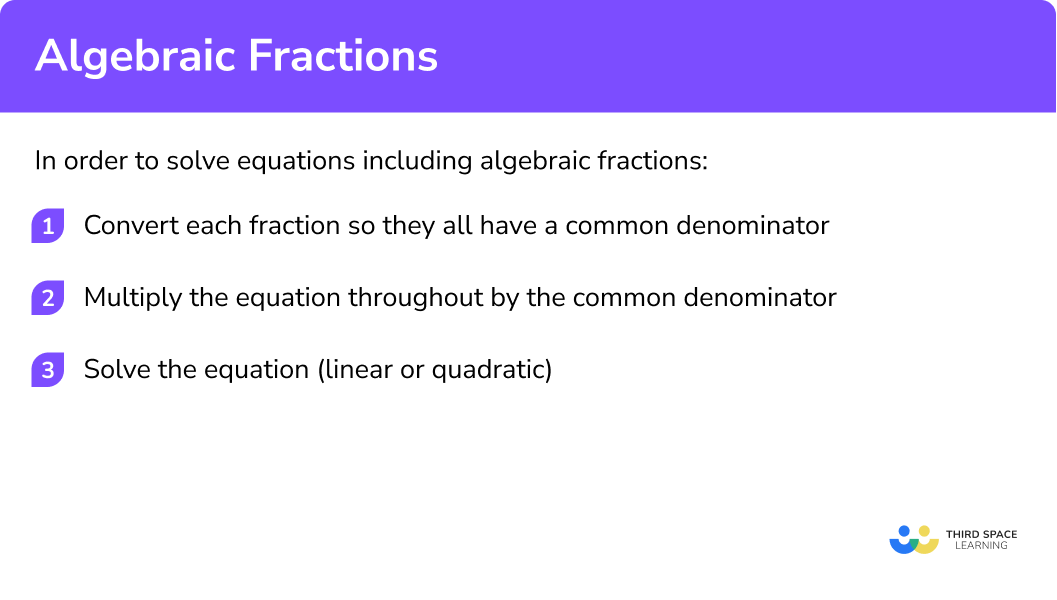# Algebraic Fractions

Here we will learn about algebraic fractions, including operations with fractions, and solving linear and quadratic equations written in the form of algebraic fractions.

There are also algebraic fractions worksheets based on Edexcel, AQA and OCR exam questions, along with further guidance on where to go next if you’re still stuck.

## What are algebraic fractions?

Algebraic fractions are fractions that contain at least one variable.

The following algebraic expressions are examples of algebraic fractions:

x is the numerator:

Both the numerator and the denominator contain an x term:

An expression in    terms of x is the    denominator:

Both the numerator    and the    denominator    contain an    expression with x :

The numerator is a multiple of x :

The numerator and the denominator are    quadratic expressions:

The main aim of this lesson is to understand how to solve equations that include algebraic fractions.

All the examples above are expressions whereas the examples below are equations as we can find specific values for x for each example to solve the equation.

E.g.

One step equation:

A separate constant term:

A linear equation:

A second fraction:

Double brackets, difference of two    squares and simultaneous equations:

It is important to be able to simplify algebraic fractions into their simplest form. If you need to practice this or need a quick refresher, see the lesson on simplifying algebraic fractions for further information.

Step-by-step guide: Simplifying algebraic fractions

### What are algebraic fractions?## How to solve equations including algebraic fractions

We need to be able to solve equations including algebraic fractions.

Let’s look at a simple example when \frac{8}{x}=2 .

Here, the denominator of the fraction contains the variable, so we first need to get the variable out of the denominator.

Rearranging the equation by multiplying both sides by x and then dividing by 2, we get the value of x=4.

We can substitute this into the original equation to prove that the answer is correct.

Here, \frac{8}{4}=2 so we have the correct answer.

We shall now consider more complicated cases when equations involve algebraic fractions.

In order to solve equations including algebraic fractions.

1. Convert each fraction so they all have a common denominator.
2. Multiply the equation throughout by the common denominator.
3. Solve the equation (linear or quadratic).

### Explain how to solve equations including algebraic fractions## Algebraic fractions examples

### Example 1: Equation with one fraction

Solve the equation

\frac{2x-1}{3}+x=3

1. Convert each fraction so they all have a common denominator.

Here, we only have one fraction and so we do not need to convert any other term into a fraction.

2Multiply the equation throughout by the common denominator.

Multiplying the equation throughout by 3 (the denominator of the fractional term), we get

Make sure that you multiply every term in the equation by 3 .

3Solve the equation (linear or quadratic).

You can check your solution by substituting the value for x into the original equation and evaluating it.

Here,

### Example 2: Equation with two fractions

Solve the equation

\frac{x+1}{2}+\frac{x+3}{5}=6

Convert each fraction so they all have a common denominator.

Multiply the equation throughout by the common denominator.

Solve the equation (linear or quadratic).

### Example 3: Equation with x in the denominator

Solve the equation

3-\frac{5}{x+1}=1

Convert each fraction so they all have a common denominator.

Multiply the equation throughout by the common denominator.

Solve the equation (linear or quadratic).

### Example 4: Equation with three fractions

Solve the equation

\frac{1}{x}+\frac{1}{2x}+\frac{1}{3x}=11

Convert each fraction so they all have a common denominator.

Multiply the equation throughout by the common denominator.

Solve the equation (linear or quadratic).

### Example 5: Denominators are expressions in terms of x

Solve the equation

\frac{x+1}{x+2}+\frac{3}{x-4}=1

Convert each fraction so they all have a common denominator.

Multiply the equation throughout by the common denominator.

Solve the equation (linear or quadratic).

### Example 6: Equation including a quadratic

Solve the equation

\frac{9x+4}{x}=-2x

Convert each fraction so they all have a common denominator.

Multiply the equation throughout by the common denominator.

Solve the equation (linear or quadratic).

### Common misconceptions

• Multiplying the numerator by the denominator

Let us look at example 2 . When multiplying throughout by 10 to remove the denominator from each fraction, the numerator has also been multiplied by 10 .

This means that the fractions have been multiplied by 100 , instead of 10 , leaving the next line of work to be incorrect.

• Ignoring the denominators

When given an equation including an algebraic fraction, if the denominators are ignored the question will be answered incorrectly.

• Not multiplying all terms by the denominator

When rearranging an equation, the denominator is moved to the other side of the equals sign, instead of each term being multiplied by it. You must remember to multiply throughout by any value, not just the opposite side of the equals sign.

• Simplifying fractions incorrectly (1)

When adding two fractions, the denominator must be the same. A common misconception for adding two fractions is to add the numerators and the denominators together because this method is emphasised when looking at multiplying fractions.

• Simplifying fractions incorrectly (2)

Seeing the same term on the numerator and denominator allow the misconception that they can both be cancelled.

### Practice algebraic fractions questions

1. Solve the equation

\frac{x+5}{3}+x=7

x=4x=1x=8x=32. Solve the equation

\frac{x+5}{2}+\frac{5+x}{2}=50

x=20x=7.5x=45x=22.53. Solve the equation

7+\frac{6x+4}{8}=2x

x=6x=1.1x=-\frac{39}{46}x=04. Solve the equation

\frac{5}{x}+\frac{2x}{5}=3

x=-1x=2.5x=\sqrt{11}x=05. Solve the equation

\frac{6-x}{4}+\frac{12}{4-x}=4

x=14x=\frac{14}{3}x=-5\pm\sqrt{31}x=-26. Solve the equation

\frac{5}{8+x}+\frac{6-x}{9}=2

x=-3x=\frac{23}{3}x=-1\pm2\sqrt{23}x=-\frac{23}{3}### Algebraic fractions GCSE questions

1. Arron earns £40 per hour. One day, he receives a bonus of £5 . He shares this day’s earnings equally with his brother and sister. If he gives away £190 , how many hours did Arron work that day?

(2 marks)

2(\frac{40x+5}{3})=190

(1)

x=7

(1)

2. Use the quadratic formula to solve the equation

\frac{2x}{x+3} + \frac{5x}{x+2} = 4

(6 marks)

2x(x+2)+5x(x+3)=4(x+3)(x+2) or equivalent

(1)

7x^{2}+19x=4x^{2}+20x+24

(1)

3x^{2}-x-24=0

(1)

x=\frac{-1\pm\sqrt{1^{2}-4\times1\times{-24}}}{2\times{3}}

(1)

x=\frac{1\pm{17}}{6}

(1)

x=3 or x=-\frac{8}{3}

(1)

3. Two shapes given below have the same area. Calculate the value for x .(7 marks)

Area of Triangle = \frac{2(x+8)}{2}=x+8

(1)

Area of Square = \left(\frac{3}{\sqrt{x}}\right)^{2}=\frac{9}{x}

(1)

x+8=\frac{9}{x}

(1)

x^2+8x-9=0

(1)

(x+9)(x-1)=0

(1)

x=1 or x=-9

(1)

Conclusion: x=1 only

(1)

## Learning checklist

You have now learned how to:

• use algebraic methods to solve linear equations in 1 variable (including all forms that require rearrangement)
• simplify and manipulate algebraic fractions

## Still stuck?

Prepare your KS4 students for maths GCSEs success with Third Space Learning. Weekly online one to one GCSE maths revision lessons delivered by expert maths tutors.

Find out more about our GCSE maths tuition programme.